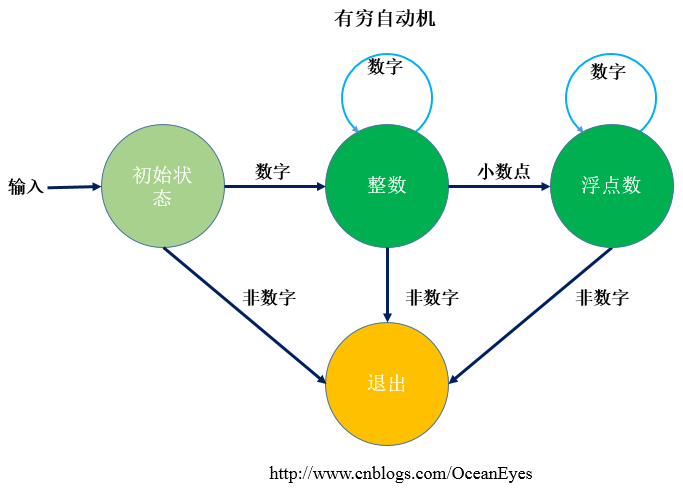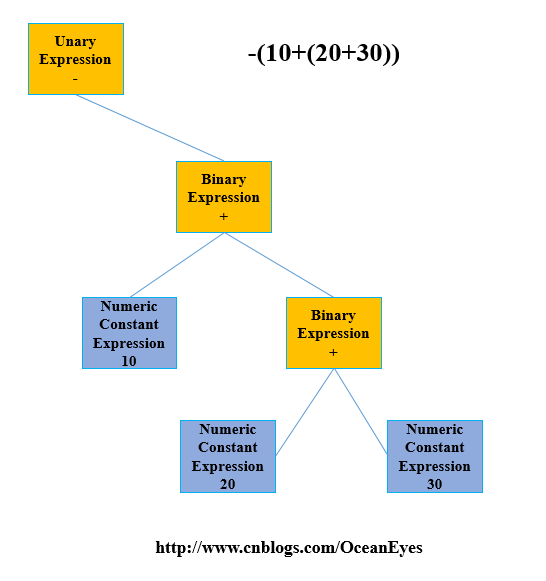# 从编译原理看一个解释器的实现

『设计模式』中有一个模式可以解释特定的语法规则，它就是解释器模式（Interpreter Pattern）。不同于常见的策略模式或者是工厂模式，解释器模式在.NET或者JDK中并不常见，而且在业务上也很少会去解释特定的语法，所以它并不被广泛使用。一个解释器可大可小，大可以是复杂的编译器，小也可以是一个简单的字符串解析，但本质上它们都是对特定的语法做出合理的解释。

### 解释器在游戏领域的应用

if (_mathExpression.GetSpecificCharByIndex(_mathExpression.CurrentIndex + 1) =='c')
{
_mathExpression.CurrentIndex += 2;
token = Token.Param;
}
else
{
_mathExpression.CurrentIndex++;
token=Token.Illegal;
}
break;
default:
if (char.IsDigit(_mathExpression.CurrentChar))
{
token = GetDigitsFromString();
}else if (char.IsLetter(_mathExpression.CurrentChar))
{
token = GetSineCosineFromString();
}
else
{
throw  new Exception("Illegal Token");
}
break;
}


3.) 值得一提的事情，怎样从字符串中获取数字，数字有两种形式：整数和小数点形式，通过有穷自动机在不同的状态间跳转并记录下数字的索引下标，直到遇到非数字退出，有穷自动机如下所示：do
{
isEndOfString = _mathExpression.IsEndOfString;
currentChar = _mathExpression.CurrentChar;

switch (_currentState)
{
case State.Init:
if (char.IsDigit(currentChar))
{
_currentState = State.Integer;
if (!isEndOfString)
{
_mathExpression.CurrentIndex++;
}
}
else
{
//Init状态非数字则退出
_currentState= State.Quit;
}
break;
case State.Integer:
if (currentChar == '.')
{
_currentState = State.Float;//输入小数点，状态转移到Float
if (!isEndOfString)
{
_mathExpression.CurrentIndex++;
}
}
else
{
if (!char.IsDigit(currentChar))//既不是数字也不是小数
{
_currentState = State.Quit;
}
else
{
if (!isEndOfString)
{
_mathExpression.CurrentIndex++;//读取下一个字符
}
}
}
break;
case State.Float:
if (!char.IsDigit(currentChar))//非数字，退出
{
_currentState = State.Quit;
}
else
{
if (!isEndOfString)
{
_mathExpression.CurrentIndex++;
}
}
break;
case State.Quit:
break;

}
} while (_currentState != State.Quit && !isEndOfString);


4.)通过语法解析器Parser构建表达式树，每个节点都是一个抽象Expression

public abstract class Expression
{
public abstract double Evaluate(Context context);
}


Expression根据类型不同有常量表达式，二元表达式，一元表达式等，一个常见的二元表达式如下：

public class BinaryExpression:Expression
{
private Expression _leftExpression;
private Expression _rightExpression;
private Operator _operator;

public BinaryExpression(Expression leftExpression,Expression righExpression,Operator op)
{
_leftExpression = leftExpression;
_rightExpression = righExpression;
_operator = op;
}
public override double Evaluate(Context context)
{
switch (_operator)
{
case Operator.Plus:
return _leftExpression.Evaluate(context) + _rightExpression.Evaluate(context);
case Operator.Minus:
return _leftExpression.Evaluate(context) - _rightExpression.Evaluate(context);
case Operator.Mul:
return _leftExpression.Evaluate(context) * _rightExpression.Evaluate(context);
case Operator.Div:
return _leftExpression.Evaluate(context) / _rightExpression.Evaluate(context);
}
return Double.NaN;
}
}


5.)到目前为止，可以说是万事俱备，只欠东风了，这个『东风』就是怎么样去构建表达式树。已知的是，一个 expr 就是一个由+或-分割开来的项（ term ）列表，而项是由x或者/分隔的因子（ factor ）列表。

expr->expr+term|expr-term|term

private Expression Expr()
{
Token old;
Expression expression = Term();
{
old = _currentToken;
_currentToken = _lexicalAnalyzer.GetToken();
Expression e1 = Expr();

}
return expression;
}


term->term x factor|term/factor|factor

private Expression Term()
{
Token old;
Expression expression = Factor();

while (_currentToken==Token.Mul || _currentToken==Token.Div)
{
old = _currentToken;
_currentToken = _lexicalAnalyzer.GetToken();

Expression e1 = Term();
expression=new BinaryExpression(expression,e1,old==Token.Mul?Operator.Mul:Operator.Div);
}

return expression;
}


factor->digit|(expr)

private Expression Factor()
{
Token token;
Expression expression;
if (_currentToken==Token.Double)
{
expression=new NumericConstant(_lexicalAnalyzer.GetDigits());
_currentToken = _lexicalAnalyzer.GetToken();
}
else if (_currentToken == Token.Param)
{
expression=new Var();
_currentToken = _lexicalAnalyzer.GetToken();
}
else if (_currentToken==Token.OParen)
{
_currentToken = _lexicalAnalyzer.GetToken();
expression = Expr();
if (_currentToken!=Token.CParen)
{
throw new Exception("Missing Closing Parenthesis\n");
}
_currentToken = _lexicalAnalyzer.GetToken();
}
{
var old = _currentToken;
_currentToken = _lexicalAnalyzer.GetToken();
expression = Factor();

}
else
{
throw new Exception("error");
}
return expression;
}### 小结###

posted @ 2017-06-18 23:25  木宛城主  阅读(9055)  评论(5编辑  收藏  举报